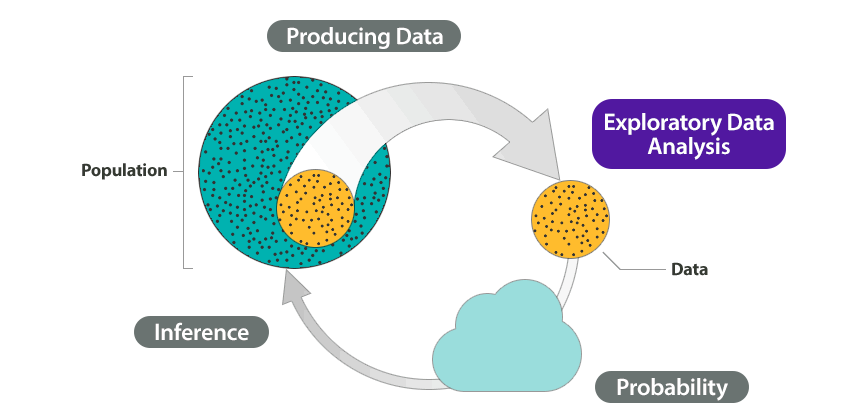# 3.1: Why It Matters- Examining Relationships- Quantitative Data

$$\newcommand{\vecs}{\overset { \rightharpoonup} {\mathbf{#1}} }$$ $$\newcommand{\vecd}{\overset{-\!-\!\rightharpoonup}{\vphantom{a}\smash {#1}}}$$$$\newcommand{\id}{\mathrm{id}}$$ $$\newcommand{\Span}{\mathrm{span}}$$ $$\newcommand{\kernel}{\mathrm{null}\,}$$ $$\newcommand{\range}{\mathrm{range}\,}$$ $$\newcommand{\RealPart}{\mathrm{Re}}$$ $$\newcommand{\ImaginaryPart}{\mathrm{Im}}$$ $$\newcommand{\Argument}{\mathrm{Arg}}$$ $$\newcommand{\norm}{\| #1 \|}$$ $$\newcommand{\inner}{\langle #1, #2 \rangle}$$ $$\newcommand{\Span}{\mathrm{span}}$$ $$\newcommand{\id}{\mathrm{id}}$$ $$\newcommand{\Span}{\mathrm{span}}$$ $$\newcommand{\kernel}{\mathrm{null}\,}$$ $$\newcommand{\range}{\mathrm{range}\,}$$ $$\newcommand{\RealPart}{\mathrm{Re}}$$ $$\newcommand{\ImaginaryPart}{\mathrm{Im}}$$ $$\newcommand{\Argument}{\mathrm{Arg}}$$ $$\newcommand{\norm}{\| #1 \|}$$ $$\newcommand{\inner}{\langle #1, #2 \rangle}$$ $$\newcommand{\Span}{\mathrm{span}}$$

## Why learn how to analyze data by examining the relationships within quantitative data?

Before we begin Examining Relationships: Quantitative Data, let’s see how the new ideas in this module relate to what we learned in the previous modules, Types of Statistical Studies and Producing Data and Summarizing Data Graphically and Numerically.

Recall the Big Picture:

We begin a statistical investigation with a research question. The investigation proceeds with the following steps:

• Produce Data: Determine what to measure, then collect the data. ← Types of Statistical Studies and Producing Data
• Explore the Data: Analyze and summarize the data. Summarizing Data Graphically and Numerically, Examining Relationships: Quantitative Data
• Draw a Conclusion: Use the data, probability, and statistical inference to draw a conclusion about the population.

Types of Statistical Studies and Producing Data focused on methods for collecting reliable data. Summarizing Data Graphically and Numerically focused on summarizing and analyzing data for a quantitative variable. In this module, we focus on summarizing and analyzing the relationship between two quantitative variables. In the Big Picture of Statistics, the material in Examining Relationships: Quantitative Data is still part of exploratory data analysis.